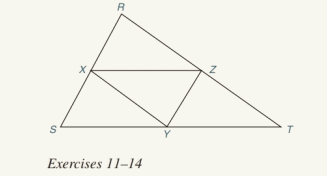Chapter 4.2, Problem 13E### Elementary Geometry for College St...

6th Edition
Daniel C. Alexander + 1 other
ISBN: 9781285195698

#### Solutions

Chapter
Section### Elementary Geometry for College St...

6th Edition
Daniel C. Alexander + 1 other
ISBN: 9781285195698
Textbook Problem
1 views

# In Exercises 11 to 14 , assume that X , Y , and Z are midpoints of the sides of ∆ R S T .For Exercise 12 to 14, see the figure for Exercise 11.If the perimeter (sum of the lengths of all three sides) of ∆ R S T is 20, what is the perimeter of ∆ X Y Z ?To determine

To find:

The perimeter of the XYZ.

Explanation

Approach:

The line segment that joins the midpoints of two sides of a triangle is parallel to the third side and has a length equal to one half the length of the third side.

Calculation:

Given,

The perimeter of RST=20 units. X, Y, Z are the midpoints of the sides of RST.

By the application of the ‘theorem the line segment that joins the midpoints of two sides of a triangle is parallel to the third side and has a length equal to one half the length of the third side’, we have

XY=12RT,

YZ=12RS, and

### Still sussing out bartleby?

Check out a sample textbook solution.

See a sample solution

#### The Solution to Your Study Problems

Bartleby provides explanations to thousands of textbook problems written by our experts, many with advanced degrees!

Get Started

#### The value of dydx for the function y=x(3x+2).

Mathematical Applications for the Management, Life, and Social Sciences

#### Evaluate the expression sin Exercises 116. 230

Finite Mathematics and Applied Calculus (MindTap Course List)

#### Sketch the polar curve. 13. r = 1 + cos 2

Single Variable Calculus: Early Transcendentals

#### For , f′(x) =

Study Guide for Stewart's Multivariable Calculus, 8th